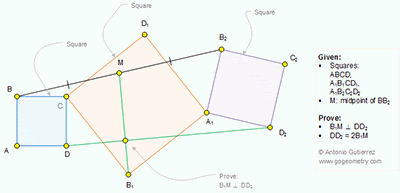Thursday, November 3, 2016

Geometry Problem 1282 Squares, Common Vertex, Midpoint, Perpendicular, 90 Degrees

Geometry Problem. Post your solution in the comment box below.
Level: Mathematics Education, High School, Honors Geometry, College.

Click the figure below to view more details of problem 1282.1.https://goo.gl/photos/QQWPcN1k1LiZB8Jt8

Let N, Q, E, F, P and R are the projection of B, D, M, B1, B2 and D2 over CA1 ( see sketch)
See sketch for positions of K, G , L and H.
Let u= D2R=A1P
v=B2P=A1R
v1=BN=CQ
u1=CN=DQ
w=FB1=FA1=FC=FD1
1. Since M is the midpoint of BB2=> E is the midpoint of NP
By observation we have EF=MG=NF-1/2.NP= ½(u1-u)
ME=1/2(v-v1)=GF
GB1= w+1/2(v-v1)
D2K=QR= v+2w-v1= 2.GB1
DK=DQ-QK=u1-u=2.MG
So MG/DK=GB1/D2K= ½
2. Triangle MGB1 similar to DKD2 ( case SAS)
So ∠ (MB1G)= ∠ (DD2K)=> D2,L, H, B1 are cocyclic
And ∠ (B1HD2)= ∠ (B1LD2)= 90 degrees
So B1M ⊥DD2

1.Thanks Peter
Another conclusion in your step 2:
DD2 = 2(B1M)

2.BY CONGRUENCE D1D2=B2B1, BB1=DD1 AND D1D2 ┴ B2B1 , BB1 ┴ DD1
Let X be the intersection of D1D2 AND B2B1,Y be the intersection of BB1 AND DD1
THEN ( C, Y, B1, A1, X, D1 ):CONCYCLIC
∠(CYD1)=45=∠(XA1D1) => ∠(XD1A1)=∠(YB1C). SO,∠(BB1B2)=90 ISW ∠(DD1D2)=90

Triangle BB1B2 ≡ DD1D2 ( SAS )
M : triangle BB1B2 with the circumcenter
So BB2=DD2=2*MB1

Let W be the intersection of MB1 AND DD2
∠YDD2= ∠B1BB2= ∠MB1B THEN (D.Y.W.B1) CONCYCLIC => ∠DYB1=∠DWB1=90

3.I wanted to open the sketch but I couldn't so now I don't understand the solution. Could someone please help me. I have to solve this for my mathclass

4.see link below for the sketch of the problem 1282

https://photos.app.goo.gl/pN56UvkWo7gKq2n23

Peter Tran